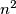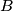### IMO Shortlist 2006 problem C4

Kvaliteta:
Avg: 3,0
Težina:
Avg: 7,0
A cake has the form of an$n$ x$n$ square composed of$n^{2}$ unit squares. Strawberries lie on some of the unit squares so that each row or column contains exactly one strawberry; call this arrangement$A$. Let$B$ be another such arrangement. Suppose that every grid rectangle with one vertex at the top left corner of the cake contains no fewer strawberries of arrangement$B$ than of arrangement$A$.

Prove that arrangement$B$ can be obtained from$A$ by performing a number of switches, defined as follows: A switch consists in selecting a grid rectangle with only two strawberries, situated at its top right corner and bottom left corner, and moving these two strawberries to the other two corners of that rectangle.
Izvor: Međunarodna matematička olimpijada, shortlist 2006